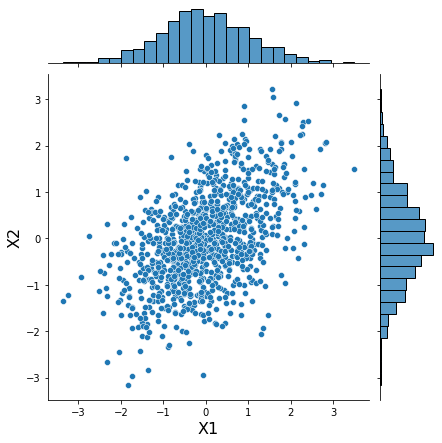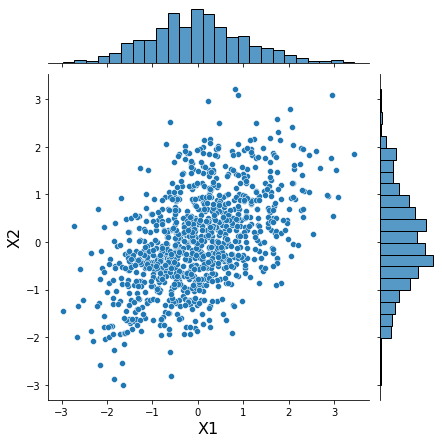# Multivariate: Vine Copulas¶

For a large selection of bivariate copula families, use pyvinecopulib’s backend. This allows for several parametric and nonparametric families to be simulated. For a complete list please see https://vinecopulib.github.io/pyvinecopulib/_generate/pyvinecopulib.BicopFamily.html.

## Import libraries¶

:

import xarray as xr
import seaborn as sns
import pyvinecopulib as pv
import synthia as syn
from scipy.stats import multivariate_normal
import warnings
warnings.filterwarnings('ignore')


## Create a sample dataset with n samples¶

:

N_SAMPLES = 1000
mvnorm = multivariate_normal(mean=[0, 0], cov=[[1, 0.5],
[0.5, 1]])
# Generate random samples from multivariate normal with correlation .5
data = xr.DataArray(mvnorm.rvs(N_SAMPLES))

h = sns.jointplot(data[:, 0], data[:, 1], kind='scatter')
h.set_axis_labels('X1', 'X2', fontsize=16);## Fit a Vine copula with pyvinecopulib’s backend¶

In this example we let pyvinecopulib choose the “best” type of copula for the type of data. In this case as we know the data was generated from a multivariate random, we could equally specify this by passing family_set to the FitControlsVinecop class (e.g. pv.FitControlsVinecop(family_set=[pv.BicopFamily.gaussian])).

:

generator = syn.CopulaDataGenerator()

parameterizer = syn.QuantileParameterizer(n_quantiles=100)

ctrl = pv.FitControlsVinecop(family_set=[pv.BicopFamily.gaussian])
generator.fit(data, copula=syn.VineCopula(controls=ctrl), parameterize_by=parameterizer)

samples = generator.generate(n_samples=N_SAMPLES)

h = sns.jointplot(samples[:, 0], samples[:, 1], kind='scatter')
h.set_axis_labels('X1', 'X2', fontsize=16);[ ]: